Win up to 100% scholarship on Aakash BYJU'S JEE/NEET courses with ABNAT Win up to 100% scholarship on Aakash BYJU'S JEE/NEET courses with ABNAT

# JEE Main 2020 Physics (Shift 1-5th Sept) Paper With Solutions

JEE Main 2020 Paper with Solutions Physics – Shift 1 will help the students to prepare efficiently for the JEE Main exams. Practising these questions will assist the candidates in increasing their solving speed along with maintaining accuracy as they are provided with detailed explanations. Some of the rigorous mistakes can be avoided by solving these question papers. This set of solutions is designed to help candidates to achieve their best scores and also to overcome their academic weaknesses by identifying gaps in exam-taking skills.

### JEE Main 2020 Physics (Shift 1-5th Sept) Paper With Solutions

September 5 Shift 1 – Physics

Question 1: Three different processes that can occur in an ideal monatomic gas are shown in the P vs V diagram. The paths are labelled as A → B, A → C and A → D. The change in internal energies during these processes are taken as EAB, EAC and EAD and the work done as WAB, WAC and WAD. The correct relation between these parameters are: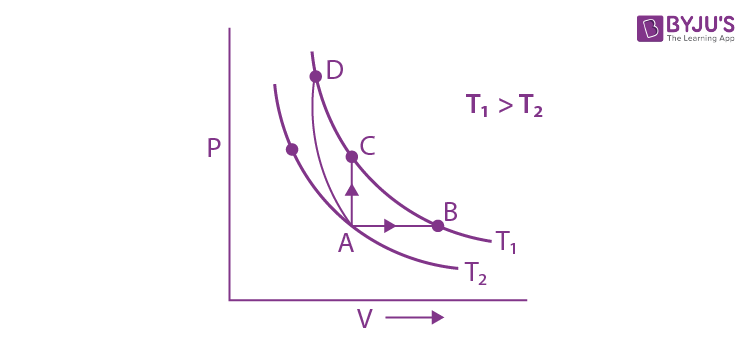1) EAB = EAC = EAD, WAB > 0, WAC = 0, WAD < 0
3) EAB < EAC < EAD, WAB > 0, WAC > WAD
4) EAB = EAC = EAD, WAB > 0, WAC = 0, WAD > 0

ΔU = nfR / 2 (Tf – Ti)

wAB > 0 (+)V ↑

wAC = 0 V constant

wAD < 0 (–) V ↓Question 2: With increasing biasing voltage of a photodiode, the photocurrent magnitude :

1) increases initially and saturates finally
2) remains constant
3) increases linearly
4) increases initially and after attaining certain value, it decreases

By theory

Question 3: A square loop of side 2a, and carrying current I, is kept in XZ plane with its centre at the origin. A long wire carrying the same current I is placed parallel to the z-axis and passing through the point (0, b, 0), (b > > a). The magnitude of the torque on the loop about the z-axis is given by:

1) 2μ0I2a3 / πb2
2) μ0I2a3 / 2πb2
3) 2μ0I2a2 / πb
4) μ0I2a3 / 2πb

M = I2 (2a)2 = 4a2 I2 (magnetic moment)

B = μ0I2 / 2πb

𝜏 = MB sin θ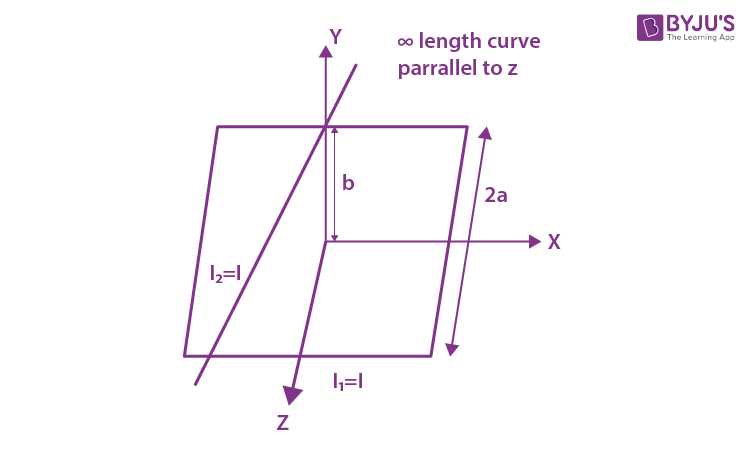θ is the angle between B and M [θ = 90°]

𝜏 = 4 (a2I2) [μ0I1 / 2πb]

𝜏 = [2μ0I1I2a2 / πb] = [2μ0I2a2 / πb]

Question 4: Assume that the displacement (s) of air is proportional to the pressure difference (Δp) created by a sound wave. Displacement(s) further depends on the speed of sound (v), the density of air (⍴) and the frequency (f). If Δp ~ 10Pa, v ~ 300 m/s, ⍴ ~ 1 kg / m3 and f ~ 1000 Hz, then s will be of the order of (take the multiplicative constant to be 1)

1) 1 mm
2) 10 mm
3) 1 / 10 mm
4) 3 / 100 mm

S0 = ΔP / βK = (ΔP) / [(⍴v2) * (ω / v)] = (ΔP) / (⍴vω) = (ΔP) / (⍴v2πf)

∴ Proportionally constant = 1

S0 = ΔP / (⍴vf)

= {10 / [1 × 300 × 1000]} m

= [10 / 300] mm

= 3 / 90

= 3 / 100 mm

Question 5: Two capacitors of capacitances C and 2C are charged to potential differences V and 2V, respectively. These are then connected in parallel in such a manner that the positive terminal of one is connected to the negative terminal of the other. The final energy of this configuration is:

1) zero
2) [9 / 2] CV2
3) [25 / 6] CV2
4) [3 / 2] CV2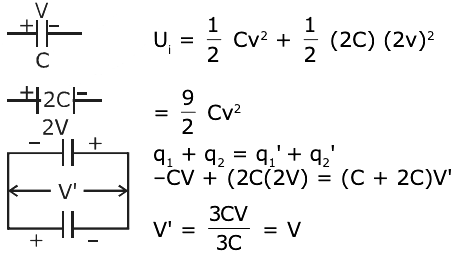Uf = (1 / 2) CV2 + (1 / 2) (2C) V2

Uf = (3 / 2) CV2

Question 6: A helicopter rises from rest on the ground vertically upwards with a constant acceleration g. A food packet is dropped from the helicopter when it is at a height h. The time taken by the packet to reach the ground is close to [g is the acceleration due to gravity]:

1) t = 3.4 √(h / g)
2) t = √(2h / 3g)
3) t = (2 / 3) √(h / g)
4) t = 1.8 √(h / g)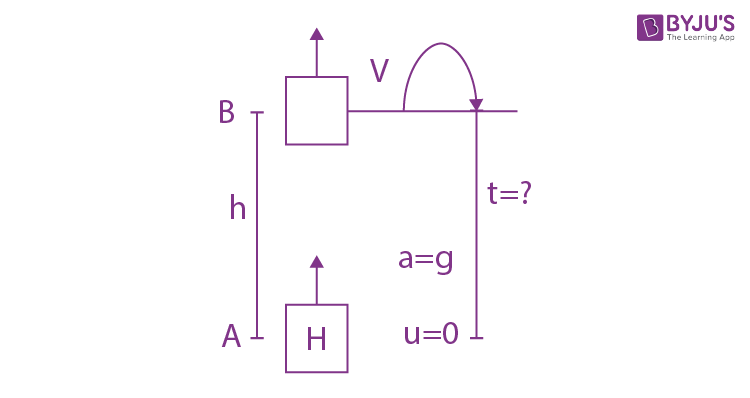VB2 = 02 + 2gh

VB = √2gh

– h = (VB) t – (1 / 2) gt2

– h = √2gh * t – (1 / 2) gt2

gt2 – 2 √2gh * t – 2h = 0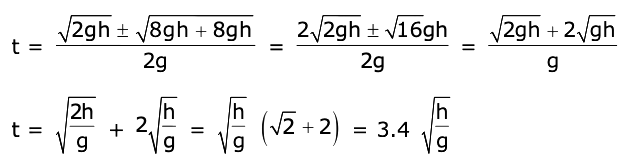Question 7: A bullet of mass 5 g, travelling with a speed of 210 m/s, strikes a fixed wooden target. One half of its kinetic energy is converted into heat in the bullet while the other half is converted into heat in the wood. The rise of temperature of the bullet if the specific heat of its material is 0.030 cal/(g – °C) (1 cal = 4.2 × 107 ergs) close to:

1) 38.4°C
2) 87.5°C
3) 83.3°C
4)119.2°C

[(1 / 2) mv2] × (1 / 2) = msΔT s = 0.03 cal/gm°C

v2 / 4 = 126 × ΔT = [[0.03 × 4.2J] / 10-3]

v2 = 4 × 126 × ΔT = 126 J/kgoC

(210)2 = 4 × 126 × ΔT

210 × 210 = 4 × 126 × ΔT

44100 = 504 × ΔT

ΔT = 44100 / 504 = 87.5°C

Question 8: A wheel is rotating freely with an angular speed on a shaft. The moment of inertia of the wheel is I and the moment of inertia of the shaft is negligible. Another wheel of the moment of inertia 3I initially at rest is suddenly coupled to the same shaft. The resultant fractional loss in the kinetic energy of the system is:

1) 3 / 4
2) 0
3) 5 / 6
4) 1 / 4

ki = (1 / 2) Iω2

kf = (1 / 2) 4I (ω’)2

= 2I (ω / 4)2 = (1 / 8) Iω2

A.M.C

Iω = (I + 3I) ω’

ω’ = Iω / 4I = ω / 4

= [Ki – Kf] / [Ki] ⇒ [{(1 / 2) Iω2 – (1 / 8) Iω2} / {(1 / 2) Iω2}]

= (3 / 8) Iω2 / (1 / 2) Iω2

= 3 / 4

Question 9: A balloon is moving up in air vertically above a point A on the ground. When it is at a height h1, a girl standing at a distance d(point B) from A (see figure) sees it at an angle 45° with respect to the vertical. When the balloon climbs up a further height h2, it is seen at an angle 60° with respect to the vertical if the girl moves further by a distance 2.464 d (point C). Then the height h2 is (given tan 30° = 0.5774):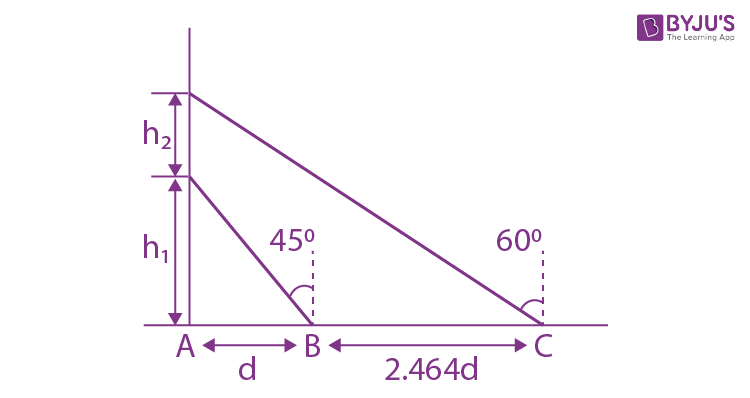1) 0.464 d
2) d
3) 0.732 d
4) 1.464 d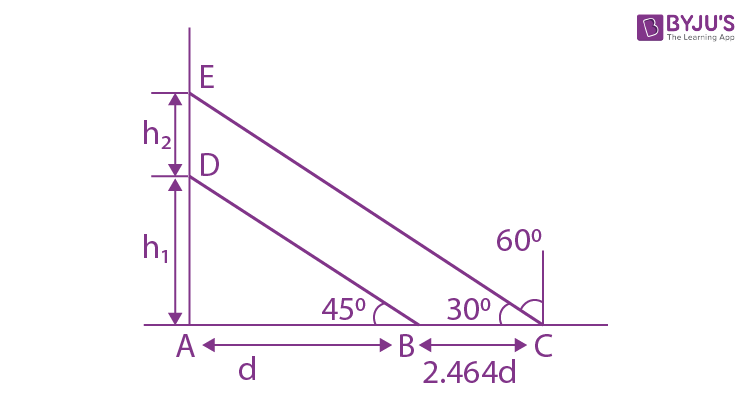△ABD

tan 45 = h1 / d

⇒ 1 = h1 / d ⇒ h1 = d

△ACE

tan 30 = [h1 + h2] / [d + 2.464d]

0.5774 = [d + h2] / 3.464d

d + h2 = 0.5774 × 3.464 × d

h2 = 2.0001136d – d

h2 = 2.000d – d = d

Question 10: An electrical power line, having a total resistance of 2Ω, delivers 1 kW at 220 V. The efficiency of the transmission line is approximately:

1) 72%
2) 91 %
3) 85%
4) 96%

𝜂 = {[Pout] / [Pout + Ploss]} * 100

I = P / V = 100 / 220 = (5 / 11) A

Ploss = I2R

= (50 / 11)2 * 2 = 41.322

𝜂 = { / [1000 + 41.322]} * 100

𝜂 = 96%

Question 11: Activities of three radioactive substances A, B and C are represented by the curves A, B and C, in the figure. Then their half-lives T1/2 (A) : T1/2 (B) : T1/2 (C) are in the ratio: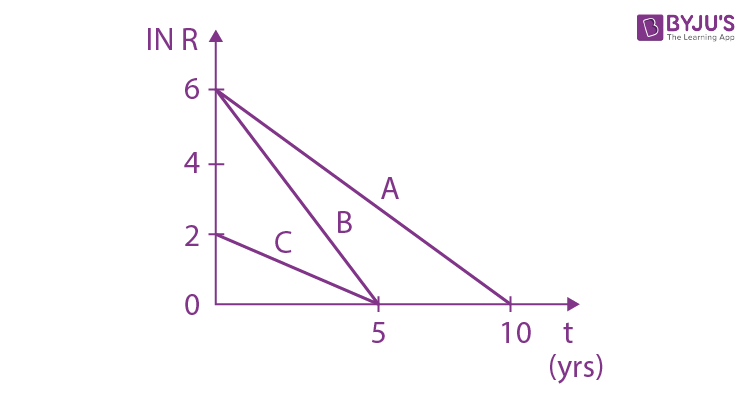1) 3 : 2 : 1
2) 2 : 1 : 1
3) 4 : 3 : 1
4) 2 : 1 : 3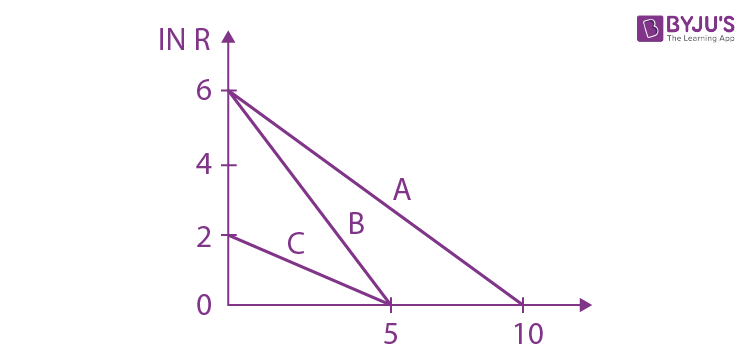R = R0e-λt

(λ = slope of the graph)

λA = (6 / 10) = (ln 2) / TA

λB = 6 / 5 = (ln 2) / TB

λC = 2 / 5 = (ln 2) / TC

TA = (5 / 3) ln 2

TB = (5 / 6) ln 2

TC = (5 / 2) ln 2

T(A) : T(B) : T(C) = (1 / 3) : (1 / 6) : (1 / 2)

= 2 : 1 : 3

Question 12: The value of the acceleration due to gravity is g1 at a height h = R / 2 (R = radius of the earth) from the surface of the earth. It is again equal to g1 at a depth d below the surface of the earth. The ratio (d / R) equals :

1) 4 / 9
2) 1 / 3
3) 5 / 9
4) 7 / 9

gat high = gat depth

gsurface = GM / R2

g (1 – [d / R]) = GM / (R + h)2

g (1 – [d / R]) = GM / [R2 (1 + (h / R))2] = g / (1 + [R / 2R])2 = 4g / 9

d / R = 1 – (4 / 9) = 5 / 9

Question 13: A hollow spherical shell at outer radius R floats just submerged under the water surface. The inner radius of the shell is r. If the specific gravity of the shell material is 27 / 8 w.r.t water, the value of r is:

1) 4 / 9 R
2) 8 / 9 R
3) 1 / 3 R
4) 2 / 3 R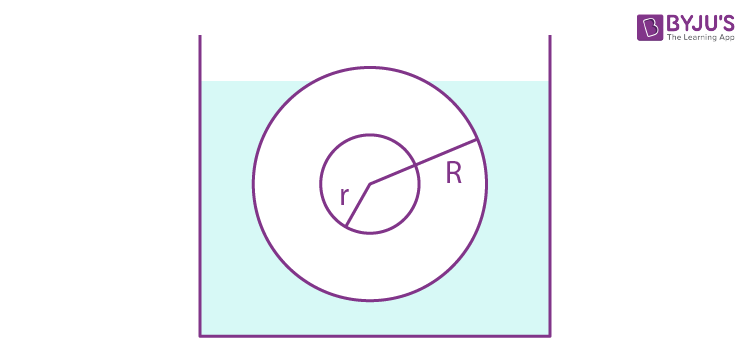FB = mg

lVbodyg = ⍴BVbg

(displaced (where mater present)

water)

(4 / 3) πR3 = [⍴B / ⍴l] [(4 / 3) πR3 – (4 / 3) πr3]

R3 = (27 / 8) (R3 – r3)

(8 / 27) R3 = (R3 – r3) ⇒ r3 = R3 – [8R3 / 27] = [19 / 27] R3

r = [(19) / 3] R ≈ 0.88 ≈ (8 / 9) R

Question 14: In a resonance tube experiment when the tube is filled with water up to a height of 17.0 cm from the bottom, it resonates with a given tuning fork. When the water level is raised the next resonance with the same tuning fork occurs at a height of 24.5 cm. If the velocity of sound in air is 330 m/s, the tuning fork frequency is:

1) 2200 Hz
2) 550 Hz
3) 3300 Hz
4) 1100 Hz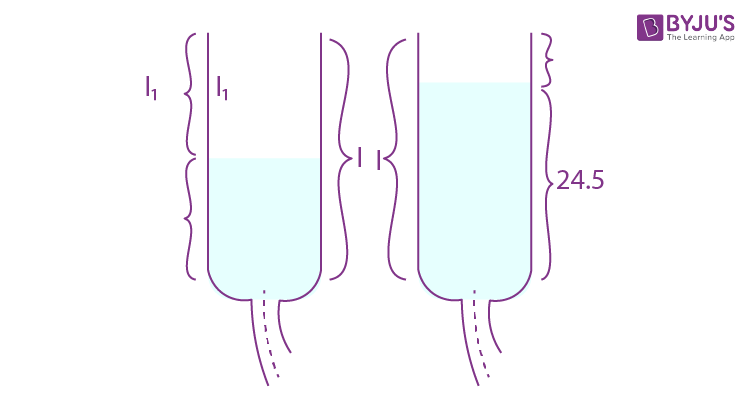l1 = 17

l2 = 24.5

v = 2f (l1 – l2)

330 = 2 x f (24 – 17) x 10–2

165 = f × 7.5 × 10–2

f = [165 × 100] / 7.5

f = 2200 Hz

Question 15: A solid sphere of radius R carries a charge Q + q distributed uniformly over its volume. A very small point-like piece of it of mass m gets detached from the bottom of the sphere and falls down vertically under gravity. This piece carries charge q. If it acquires a speed v when it has fallen through a vertical height y (see figure), then : (assume the remaining portion to be spherical).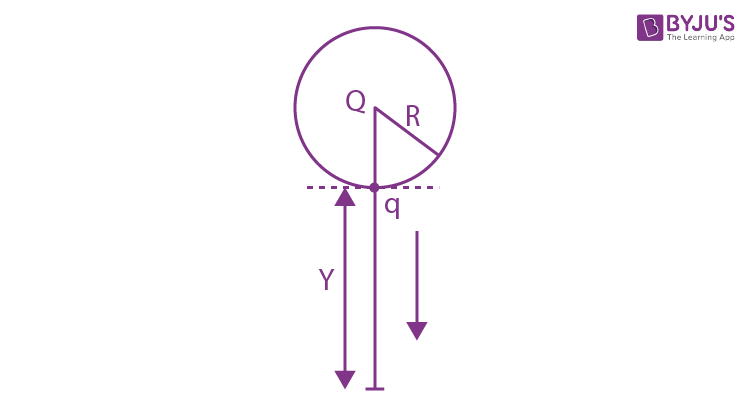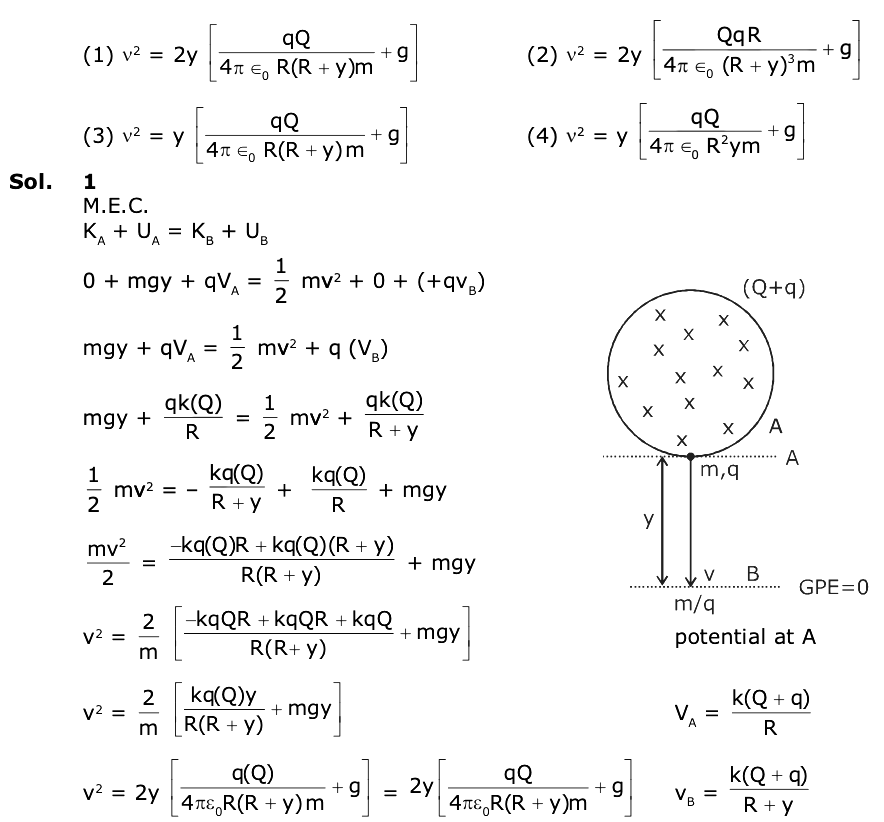Question 16: A galvanometer of resistance G is converted into a voltmeter of range 0 – 1V by connecting a resistance R1 in series with it. The additional resistance that should be connected in series with R1 to increase the range of the voltmeter to 0 – 2V will be:

1) G
2) R1
3) R1 – G
4) R1 + G

V = I (R1 + G)

(1 / 2) = [(I (R1 + G)] —- (i) / [(I (R1 + R2 + G)] —- (ii)

(1 / 2) = [(R1 + G)] / [(R1 + R2 + G)]

R1 + R2 + G = 2R1 + 2G

R2 = R1 + G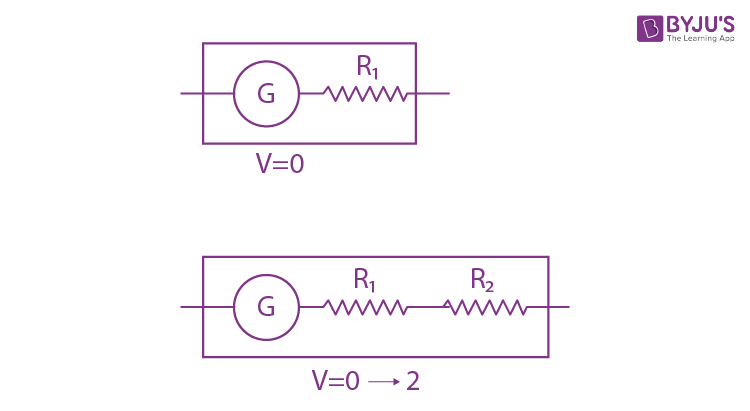Question 17: Number of molecules in a volume of 4 cm3 of a perfect monatomic gas at some temperature T and at a pressure of 2 cm of mercury is close to? (Given, mean kinetic energy of a molecule (at T) is 4 × 10–14 erg, g = 980 cm/s2, density of mercury = 13.6 g/cm3)

1) 4.0 × 1018
2) 4.0 × 106
3) 5.8 × 1016
4) 5.8 × 1018

$$\begin{array}{l}n = \frac{PV}{RT},\: \frac{3}{2}kT = 4 \times 10^{-14}\end{array}$$
$$\begin{array}{l}N = \frac{PV}{RT} \times Na\end{array}$$
$$\begin{array}{l}\frac{2\times 13.6\times980 \times 4}{\frac{8}{3}\times 10^{-14}}\end{array}$$

N = 3.99 x 1018

or N = 4.0 x 1018

Question 18: An electron is constrained to move along the y-axis with a speed of 0.1 c (c is the speed of light) in the presence of electromagnetic wave, whose electric field is E = 30j sin (1.5 × 107 t –5×10–2x) V/m. The maximum magnetic force experienced by the electron will be : (given c = 3 × 108 ms–1 and electron charge = 1.6 × 10–19C)

1) 2.4 × 10–18 N
2) 4.8 × 10–19 N
3) 3.2 × 10–18 N
4) 1.6 × 10–19 N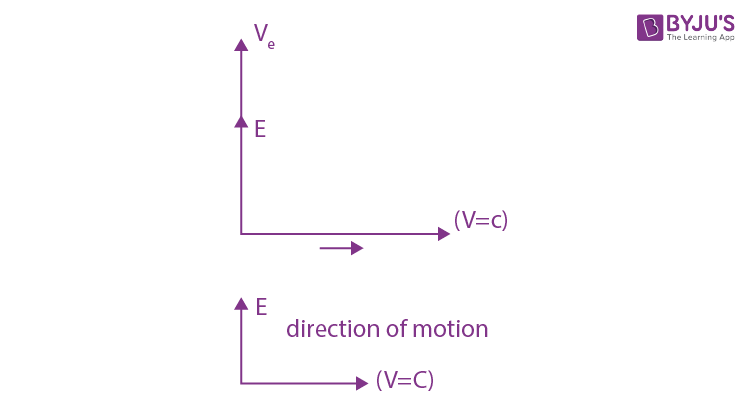ve = 0.1C along y-axis direction of emwave – along (x)

E = v × B

E = CB ⇒ B = E / C

∴ force on e will be max.

If B is ⟂ to y-along z-axis

[∵ E also ⟂ B, B also ⟂ to the direction of motion of wave]

∴ B → along Bz (–z) as

B = [30 / C] sin (1.5 × 107 t – 5 × 10–2x)

Bmax = 30 / [3 × 108] = 10–7 T

θ = 90 between ve & B so Fmax =qvB

Fmax = e × (0.1 × C) × [30 / C]

= 1.6 × 10–19 × 3

Fmax = 4.8 × 10–19 N

Question 19: For a concave lens of focal length f, the relationship between object and image distances u and v, respectively, from its pole can best be represented by (u = is the reference line) :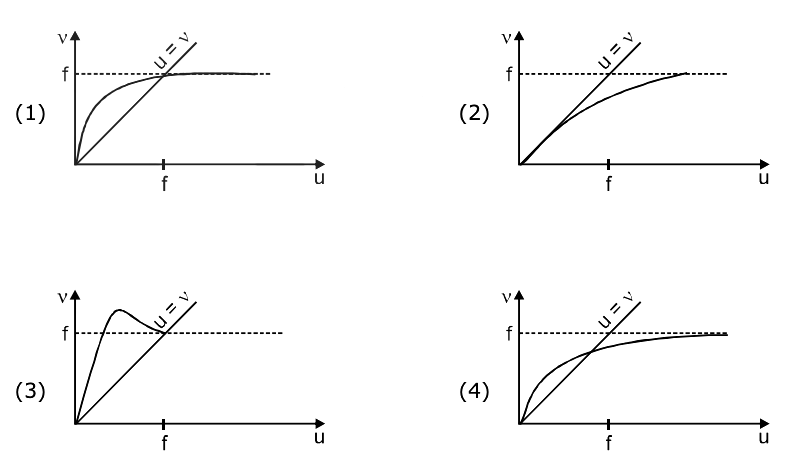From the lens formula,

(1 / v) – (1 / u) = 1 / f

v = [uf] / [u + f]

At reference line (u = v)

u = 0

At u = ∞

v = f for exact idea of curve slope at u = 0 will be 450

[dv / du]u=0 = 1

Question 20: A physical quantity z depends on four observables a, b, c and d, as z = [a2b2/3 / √c d3]. The percentages of error in the measurement of a, b, c and d are 2% , 1.5%, 4% and 2.5% respectively. The percentage of error in z is :

1) 16.5 %
2) 12.25 %
3) 13.5%
4) 14.5%

z = a2b2/3c–1/2d–3

100 × [dz / z] = [2 (da / a) + (2 / 3) (db / b) + (1 / 2) (dc / c) + 3 d (d) / d] × 100

% error in z

= [2 × 2 + (2 / 3) × 1.5 + (1 / 2) × 4 + 3 × 2.5]%

= 4 + 1 + 2 + 7.5

= 14.5 %

Question 21: A particle of mass 200 Me V/c2 collides with a hydrogen atom at rest. Soon after the collision, the particle comes to rest, and the atom recoils and goes to its first excited state. The initial kinetic energy of the particle (in eV) is N / 4. The value of N is : (Given the mass of the hydrogen atom to be 1 GeV/c2)_____.

mH = 1GeVC2 = 1000 MeV/C2, mparticle = 200 meV/c2 = m∴ mv0 + 0 = 0 + 5 mV’ ⇒ v’ = v0 / 5

Loss in KE

= (1 / 2) mv02 – (1 / 2) (5m) (v0 / 5)2

= (4 / 5) (mv02 / 2) = (4 / 5) k

(4 / 5) k = 10.2

k = 12.75 eV = 12.75 / 100 = 51 / 4

n = 51

Question 22: Two concentric circular coils, C1 and C2, are placed in the XY plane. C1 has 500 turns, and a radius of 1 cm. C2 has 200 turns and a radius of 20 cm. C2 carries a time-dependent current I (t) = (5t2 – 2t + 3) A where t is in s. The emf induced in C1 (in mV), at the instant t = 1s is 4 / x. The value of x is_____.

B2 = μ0I2N2 / 2R2

ɸ = N1B2πR12 = N1N2 0I / 2R2) πR12

e = dɸ / dt

ɸ = [500 × 200 × 4π × 10-7 × (5t2 – 2t – 3)π × (10-2)2] / [2 × 20 × 10-2] [105 × 4π2 × 10-7 (5t2 – 2t + 3) × 10-4] / [40 × 10-2]

ɸ = (5t2 – 2t + 3) × 10-4

e = |dɸ / dt| = (10t – 2) × 10–4

t = 1sec

e = 8 × 10–4 = 0.8 mV = 8 / 10 = 4 / 5

x = 5

Question 23: A beam of electrons of energy E scatters from a target having atomic spacing of 1Å. The first maximum intensity occurs at θ = 60° Then E (in eV) is ______. (Planck constant h = 6.64 × 10–34 Js, 1 eV = 1.6 × 10–19 J, electron mass m = 9.1 × 10–31 kg)

2d sin θ = nλ = 1 × √(150 / v) × 10-10, θ = 90° – (ɸ / 2)

2 × 10-10 × sin 60 = √(150 / v) × 10-10, θ = 90° – (60 / 2) = 60

2 × √3 / 2 = √(150 / v)

V = 150 / 3 = 50 volt

E = qv = ev = 50ev

Question 24: A compound microscope consists of an objective lens of focal length 1 cm and an eyepiece of focal length 5 cm with a separation of 10 cm. The distance between an object and the objective lens, at which the strain on the eye is minimum is n / 40 cm. The value of n is _____.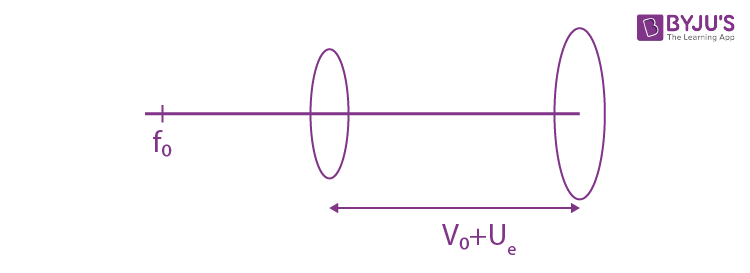f0 = 1cm, fe = 5 cm, u0 = ?

final image at (∞)

(ve = ∞)

v0 + ue = 10cm …(i) L = v0 + ue = 10 cm

v0 + 5 = 10 (1 / ve) – (1 / ue) = 1 / fe

v0 = 5 cm (1 / ∞) – (1 / ue) = 1 / 5

(1 / v0) – (1 / u0) = (1 / f0) ue = –5cm

(1 / 5) – (1 / u0) = 1 / 1 |ue| = 5

(1 / u0) = [1 / 5] – 1 = – 4 / 5 ⇒ u0 = – 4 / 5

|u0| = 5 / 4 = 50 / 40 = n / 40

n = 50

Question 25: A force F = (i + 2j + 3k) N acts at a point (4i + 3j – k) m. Then the magnitude of torque about the point (i + 2j + k) m will be √x N – m. The value of x is ______.

𝜏 = r × F = (3i + j – 2k) (i + 2j + 3k)

=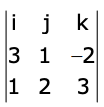= i (3 + 4) – j (9 + 2) + k (6 – 1)

𝜏 = 7i – 11j + 5k

|𝜏| = √49 + 121+ 25 = √195

x = 195